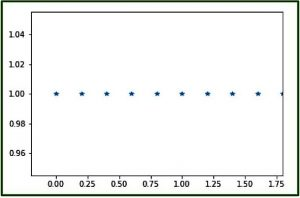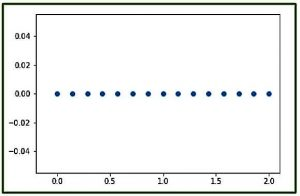Open in App
Not now

# numpy.linspace() in Python

• Difficulty Level : Easy
• Last Updated : 19 May, 2022

The numpy.linspace() function returns number spaces evenly w.r.t interval. Similar to numpy.arange() function but instead of step it uses sample number.
Syntax :

```numpy.linspace(start,
stop,
num = 50,
endpoint = True,
retstep = False,
dtype = None)```

Parameters :

```-> start  : [optional] start of interval range. By default start = 0
-> stop   : end of interval range
-> restep : If True, return (samples, step). By default restep = False
-> num    : [int, optional] No. of samples to generate
-> dtype  : type of output array```

Return :

```-> ndarray
-> step : [float, optional], if restep = True```

Code 1 : Explaining linspace function

## Python

 `# Python Programming illustrating``# numpy.linspace method` `import` `numpy as geek` `# restep set to True``print``(``"B\n"``, geek.linspace(``2.0``, ``3.0``, num``=``5``, retstep``=``True``), ``"\n"``)` `# To evaluate sin() in long range``x ``=` `geek.linspace(``0``, ``2``, ``10``)``print``(``"A\n"``, geek.sin(x))`

Output :

```B
(array([ 2.  ,  2.25,  2.5 ,  2.75,  3.  ]), 0.25)

A
[ 0.          0.22039774  0.42995636  0.6183698   0.77637192  0.8961922
0.9719379   0.99988386  0.9786557   0.90929743]```

Code 2 : Graphical Representation of numpy.linspace() using matplotlib module – pylab

## Python

 `# Graphical Representation of numpy.linspace()``import` `numpy as geek``import` `pylab as p` `# Start = 0``# End = 2``# Samples to generate = 10``x1 ``=` `geek.linspace(``0``, ``2``, ``10``, endpoint ``=` `False``)``y1 ``=` `geek.ones(``10``)` `p.plot(x1, y1, ``'*'``)``p.xlim(``-``0.2``, ``1.8``)`

Output :Code 3 : Graphical Representation of numpy.linspace() using pylab

## Python

 `# Graphical Representation of numpy.linspace()``import` `numpy as geek``import` `pylab as p` `# Start = 0``# End = 2``# Samples to generate = 15``x1 ``=` `geek.linspace(``0``, ``2``, ``15``, endpoint ``=` `True``)``y1 ``=` `geek.zeros(``15``)` `p.plot(x1, y1, ``'o'``)``p.xlim(``-``0.2``, ``2.1``)`

Output :Note :
These NumPy-Python programs won’t run on online IDE’s, so run them on your systems to explore them
.
This article is contributed by Mohit Gupta_OMG 😀. If you like GeeksforGeeks and would like to contribute, you can also write an article using write.geeksforgeeks.org or mail your article to review-team@geeksforgeeks.org. See your article appearing on the GeeksforGeeks main page and help other Geeks.